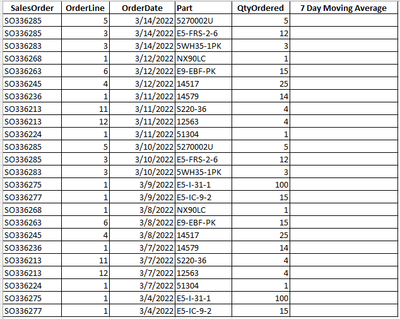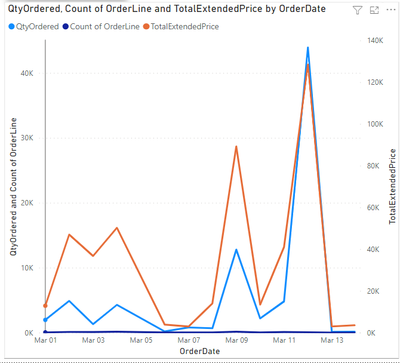cancel
Showing results for
Did you mean:Regular Visitor

## 7 day moving average

I really have zero DAX experience.  I even tried to add a column and then enter an Excel formula at first.  Of course after digging in a little I realized that it doesn't work that way in this case.  I am using direct query from a SQL database.

My query data looks like the below table - and I am trying to add the final 7 day moving average column.  In short, I need to sum the quantity ordered on each date and then calculate the 7 day moving average on those sums.  I would then add that data to the line graph below.

What is the best way to go about doing this?  In addiiton, is there a particular trainng course I can take to come up to speed?1 ACCEPTED SOLUTIONCommunity Support

Hi @dmunger ,

``Measure = CALCULATE(SUM('Table'[qtyordered]),FILTER(ALLSELECTED('Table'),'Table'[orderdate]>SELECTEDVALUE('Table'[orderdate])-7&&'Table'[orderdate]<=SELECTEDVALUE('Table'[orderdate])))/7``Best Regards,

Jay

Community Support Team _ Jay
If this post helps, then please consider Accept it as the solution
to help the other members find it.
4 REPLIES 4Regular Visitor

truptis - thank you for this.  I couldn't figure out how to modify it for my report, but that's all on me.  The measure that was suggested seems to be working great though, so I have a working model now.  Again, thank you.Community Support

Hi @dmunger ,

``Measure = CALCULATE(SUM('Table'[qtyordered]),FILTER(ALLSELECTED('Table'),'Table'[orderdate]>SELECTEDVALUE('Table'[orderdate])-7&&'Table'[orderdate]<=SELECTEDVALUE('Table'[orderdate])))/7``Best Regards,

Jay

Community Support Team _ Jay
If this post helps, then please consider Accept it as the solution
to help the other members find it.Regular Visitor

jayw - This worked perfectly, thank you!Super User

Hi @dmunger ,

Try using this:

TotalSales = Sum(Sales)

Avg Sales (MA 7 days) =
//Selecting the date in the range
VAR _LastDate =
MAX(Calendar[Date] )

VAR _Duration = 7  //Defining the duration to be considered for average calculation(k)
//For filtering the Calendar Table for the defined range
VAR_CalculationPeriod =
FILTER(
ALL(Calendar ),
AND(Calendar[Date] > _LastDate — _Duration, — the range start date
Calendar[Date] <= _LastDate — the range end date
)
)
VAR _MovingAverage =
IF (COUNTROWS ( _CalculationPeriod ) >= _Duration,
CALCULATE(AVERAGEX(Calendar,[TotalSales]), _CalculationPeriod))
RETURN
_MovingAverage

@dmunger -> Please hit the thumbs up & mark it as a solution if it works for you. Thank you.